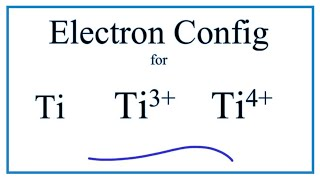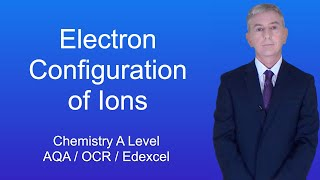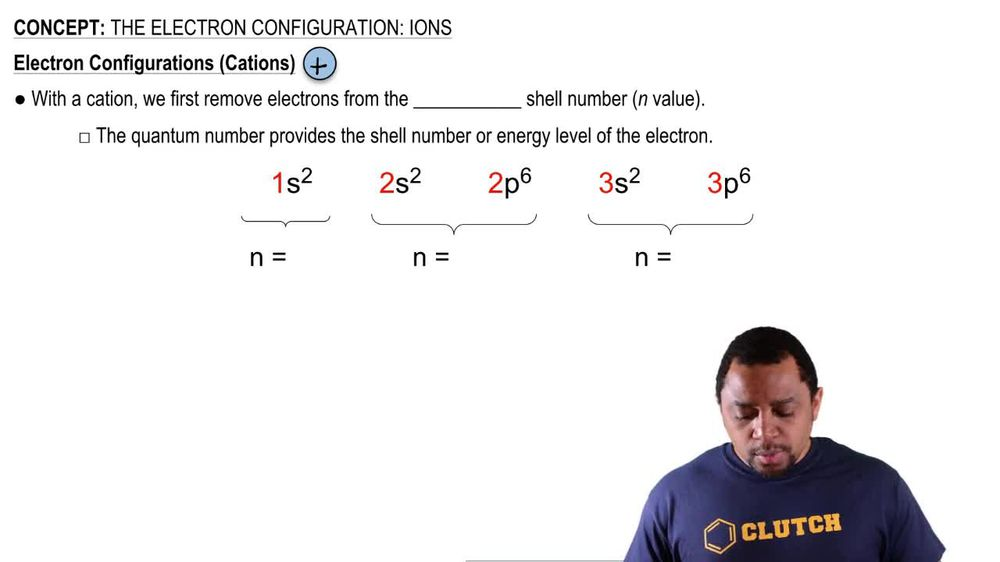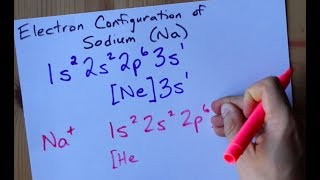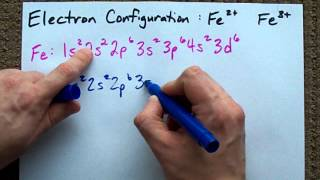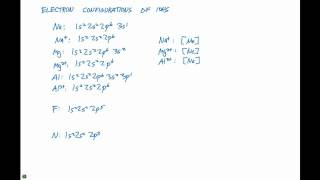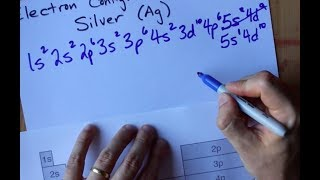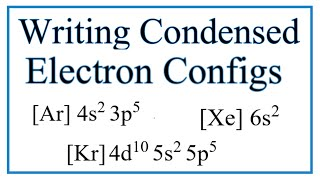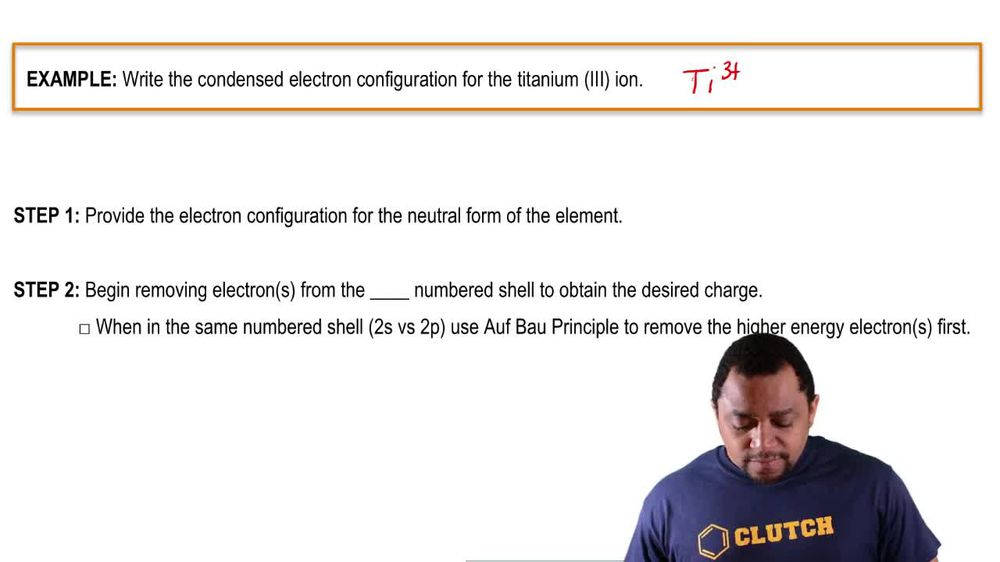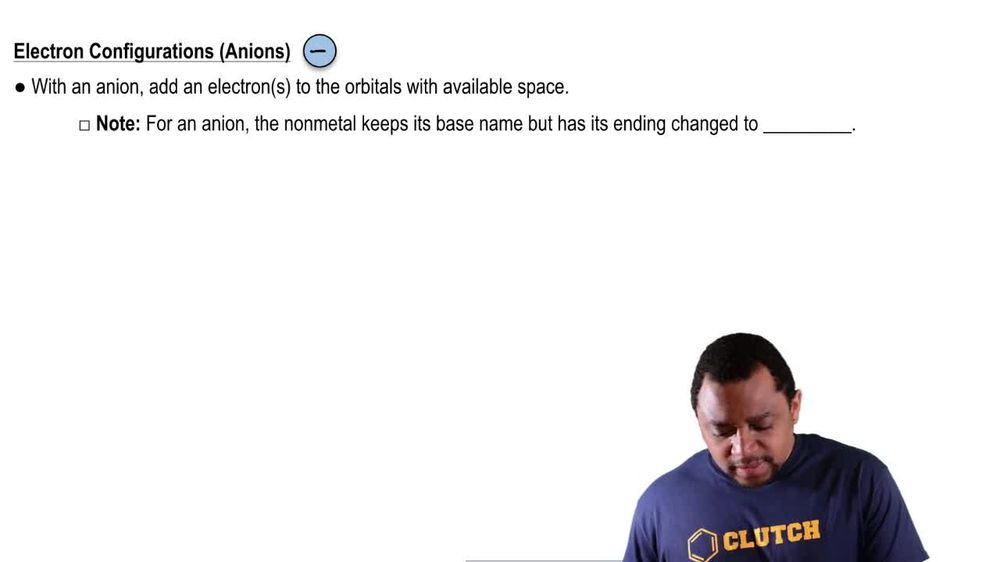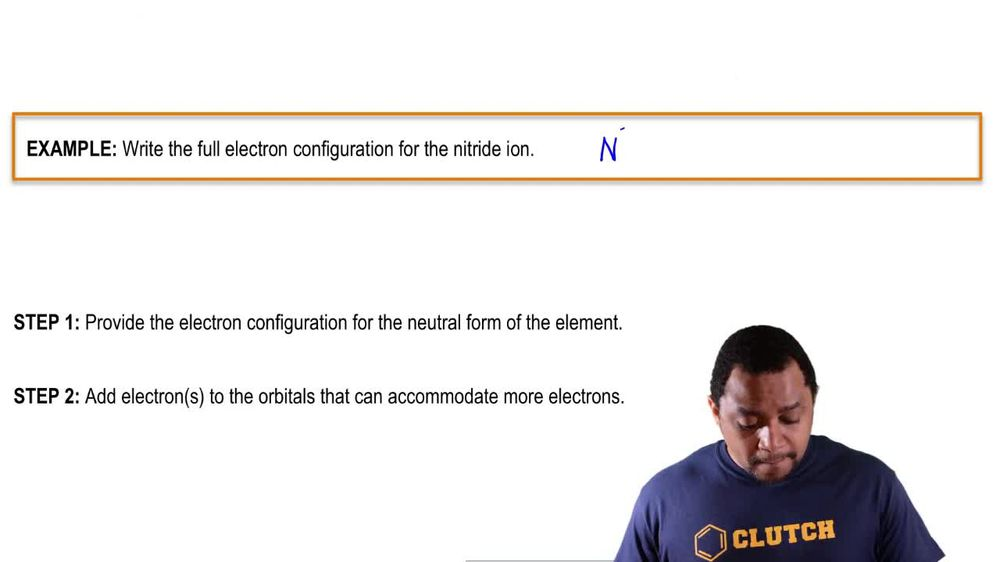Start typing, then use the up and down arrows to select an option from the list.
1. 10. Periodic Properties of the Elements2. The Electron Configuration: Ions# The Electron Configuration: Ions

by Jules Bruno
155 views
0
we'll call that when elements lose or gain electrons, they become ions were going to say here that cat ions are positive ions. I'm going to stay with the cat ion. We first remove electrons from the highest shell number and the highest shell number is connected to our principal quantum number or the end value. Now, the principal quantum number provides the shell number or energy level of the electron. So here we have one s to to us to two p 63 s, 23 p six. The number is related to the principal quantum number. N one s two would have an end value of one to us two and two P would have n values equal to two. Three s and three p would have n values equal to three. So just remember we look at the number before the sub level or sub shell letters off S P, D or F to determine our end value. And when we're removing electrons where you remove them from the orbital's that have the highest end value. But let's say we wanted to remove electrons and we only had three s and three p to choose from both of them have the highest end value. But we'd say that since three p is listed after three s, it would have more energy. And therefore, if we have to choose between three s and three p, we remove the electron first from three p. Same thing could be said between two s and two p. Since two p is listed after two s, it has more energy. And therefore, if we had to take electrons away, it come out of the two p orbital's before we even look at the two s orbital's. So just remember, first, look at the highest end value. If a set of orbital's have the same end value than the higher energy, one is the one that was listed last. That's where we order move electrons first.